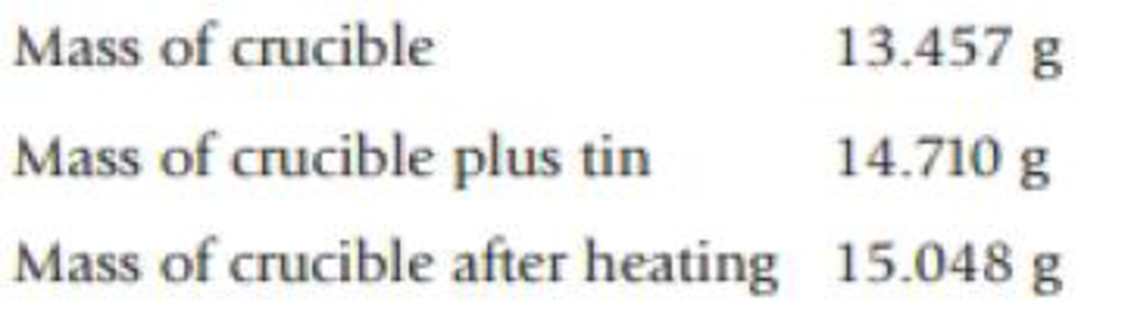Chapter 2, Problem 164IL

Chapter
Section
Textbook Problem

To find the empirical formula of tin oxide, you first react tin metal with nitric acid in a porcelain crucible. The metal is converted to tin nitrate, but, on heating the nitrate strongly, brown nitrogen dioxide gas is evolved and tin oxide is formed. In the laboratory you collect the following data:What is the empirical formula of tin oxide?

Interpretation Introduction

Interpretation: The empirical formula for tin oxide should be determined using given data under given conditions.

Concept introduction:

• Empirical formula of a compound represents the smallest whole number relative ratio of elements in that compound.
• Equation for number moles from mass and molar mass,

Numberofmoles=MassingramsMolarmass

Moles: One mole is equivalent to the mass of the substance consists same number of units equal to the atoms present in 12g of 12C.

Mass: It is the quantitative measure of a substance. The amount of matter present in substance is expressed as mass. The S.I. unit of mass is kg.

Explanation

The tin metal allowed reacting with nitric acid in a porcelain crucible. The metal is converted to tin nitrate, but on heating the nitrate strongly, brown nitrogen dioxide gas evolved and tin oxide is formed.

Given,

Massofcrucible =13.457gMassofcrucible+tin=14.710gMassofCrucibleafterheating=15.048g

Mass of tin =14.710g13.457g=1.253g

Mass of oxygen=15.048g14.710g=0

Still sussing out bartleby?

Check out a sample textbook solution.

See a sample solution

The Solution to Your Study Problems

Bartleby provides explanations to thousands of textbook problems written by our experts, many with advanced degrees!

Get Started

Find more solutions based on key concepts